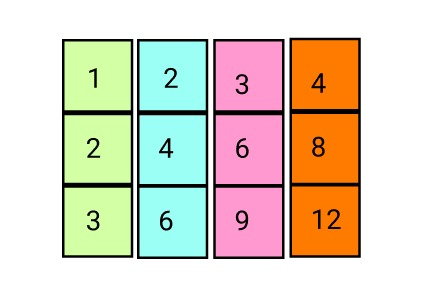Posted: 16 Mar, 2021
Difficulty: Easy

## PROBLEM STATEMENT

#### Example:

``````‘M’ is ‘3’, ‘N’ is ‘4’and ‘K’ is ‘5’. For this input, multiplication table is:
````````````So the ‘5th’ smallest number is ‘3’(‘1’, ‘2’, ‘3’, ‘3’, ‘4’, ‘4’, ‘6’, ‘6’, ‘8’, ‘9’, ‘12’)
``````

#### Input Format:

``````The first line of input contains a ‘T’ number of test cases.

In the second line, three space-separated integers ‘M’, ‘N', and ‘K' denoting height and length of the multiplication table, and ‘K’ represents the ‘Kth’ smallest number which we have to find from the multiplication table.
``````

#### Output Format:

``````For each test case, print a single line containing a single integer denoting the ‘Kth’ smallest number.

The output of each test case will be printed in a separate line.
``````
##### Note:
``````You do not need to print anything. It has already been taken care of. Just implement the given function.
``````

#### Constraints:

``````1 <= T <= 10000
1 <= M,N <= 10 ^ 7
1<= K <= M*N

Time Limit: 1 second.
``````Approach 1
• The simplest approach is that first,  we define a matrix ‘Mat’ of size ‘((M+1) * (N+1))’
• Now we make a multiplication table on the basis of a given value as each matrix element is equal to the product of its indices i.e. ‘i’ row and ‘j’ column element are equal to the ‘i * j’. So in this way filled our matrix.
• Iterate a loop ‘i’ from ‘1’ to ‘m’
• Iterate another nested loop ‘j’ from ‘1’ to ‘N’
• ‘Mat[i][j] = i * j’.
• Now we fill all the elements in our array ‘arr’ and use sorting to sort that array.
• Now we can easily find the ‘Kth’ smallest element from the array and return it as our answer.